# Numerical Implementation of Direct and Reverse Flow for Plate Heat Exchanger

Numerical Implementation of Direct and Reverse Flow for Plate Heat Exchanger
Department of Chemical Engineering and Petroleum Industries, Al-Mustaqbal University College, Hillah 51001, Babylon, Iraq

Department of Refrigeration and Air Conditioning Engineering, Al-Rafidain University College, Baghdad 10001, Iraq

Training and Workshop Center, University of Technology-Iraq, Baghdad 10001, Iraq

Corresponding Author Email:
Laith.J.Habeeb@uotechnology.edu.iq
Page:
491-500
|
DOI:
https://doi.org/10.18280/mmep.100215
11 August 2022
|
Revised:
14 November 2022
|
Accepted:
20 November 2022
|
Available online:
28 April 2023
| Citation

OPEN ACCESS

Abstract:

One of the best solutions for the heat exchange is the plate heat exchangers, which are characterized by high thermal efficiency. Where in this paper work has been done on a plate heat exchanger with dimensions appropriate to its practical application and changing the fluid exit areas to increase the surface area of exchange. And the use of more than one class to see the improvements and changes that can be analyzed and benefited from the first part represents the heat exchanger with a direct direction and the change in the number of plates. Where two sets of models were designed, the first representing the change in the number of plates, where 10, 20 and 30 plates were used to irrigate the real rate of increasing the number of plates. As for the second part, it represents the heat exchanger with a reverse path and compare them with each other. The result show that when increasing the number of plates in the plate heat exchanger, it increases the transfer area and thus increases the value of the transferred heat energy. In the case where the best transfer of heat energy is compared to 20 and 10 blades, where the exit temperature reached 308.1 K, it is noticing an increase in the transfer of thermal energy.

Keywords:

ANSYS simulation, CFD, solid works program, FEM, flow distribution, numerical study, plate heat exchanger, pressure drops

1. Introduction

2. Originality

The study of heat exchangers requires the study of correct solutions that increase the thermal efficiency of heat exchangers. Through previous research, it is noted that the study that was conducted before interfered with cracks inside the plates, and this increases the pressure difference. As for this research paper, it was centered on changing and prolonging the fluid path. To cover it the necessary time for heat exchange.

3. Methodology

One of the most prominent engineering design programs known at the present time is the solid work program, which can design engineering models with great accuracy and care. Where the design of the heat exchanger is Figure 1.

## 1.png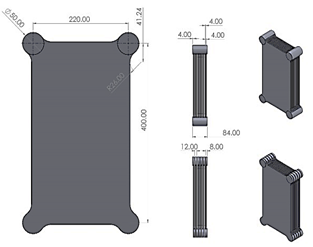Figure 1. Geometry design

Where two sets of models were designed, the first representing the change in the number of plates, where 10, 20 and 30 plates were used to irrigate the real rate of increasing the number of plates as shown in Figure 2. As for the second group, it represented the opposite direction of flow, where the entrance to the upper heat exchanger and the exit from the bottom are in one plate, and so the process is repeated until reaching the exit area.

## 2a.png(a)

## 2b.png(b)

## 2c.png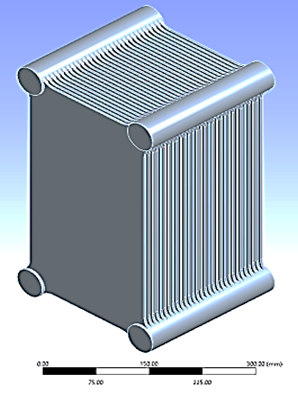(c)

The simulation process requires the work of complex algorithms to solve the matrices contained in the domain, and therefore an accurate network must be made to solve the equations. And then work the reliability of the network for a solution to reach a stable state with the results. Because of the multiplicity of models that have been simulated, it is necessary to make more than one network and more than one network reliability. In the case where the number of plates is 10, the value of the net was 3187738 when the heat at the exit area reached 308.031 K. As for the second case, when the number of plates is 20 and the network reached 6342342, then the temperature was 308,279 K. Finally, in the case where the number of plates is 30, the value of the mesh was 9519873, where the exit temperature was 308.104 K as shown in Figures 3 and 4.

## 3a.png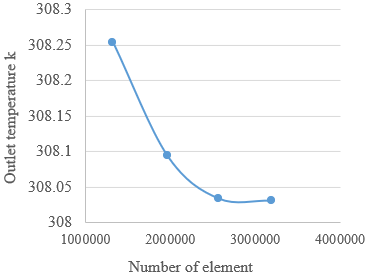(a)

## 3b.png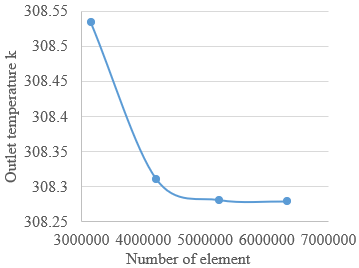(b)

## 3c.png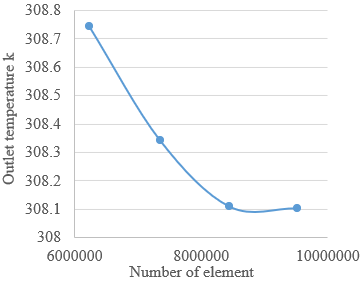(c)

## 4a.png(a)

## 4b.png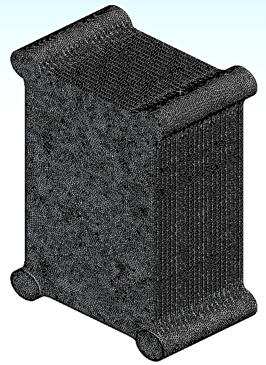(b)

## 4c.png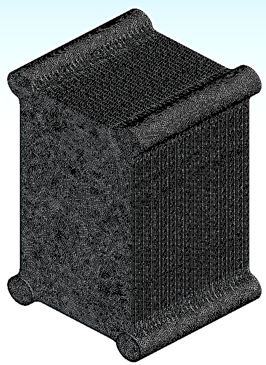(c)

Figure 4. (a) 10 blades mesh geometry; (b) 20 blades mesh geometry; (c) 30 blades mesh geometry

In order for the simulation process to take place, the appropriate settings and models for the solution must be developed to extract and compare the results. Where a constant entry velocity of 0.01 m/s was used at the entry area and a temperature of 313 K. As for the exit area, an exit pressure of 0pa was used. As for the remaining surfaces, convective heat transfer was applied to them, where the value of the heat transfer coefficient was 5 W/m.K and at an external temperature of 300 K as shown in Figure 5.

## 5a.png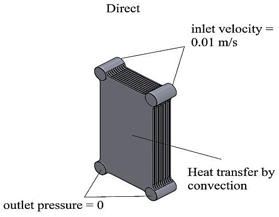(a)

## 5b.png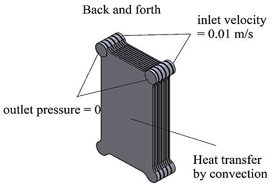(b)

Figure 5. Boundary condition of: (a) direct flow; (b) back and forth flow

4. Governing Equations

The condition for the state of movement is as per the following:

$\frac{\partial \rho}{\partial t}+\nabla \cdot(\rho \vec{v})=S_m$     (1)

In condition one it is on account of general movement, yet in condition 2 the condition is as a heading, or at least, it is in an extraordinary case, as it is given in the accompanying structure.

$\frac{\partial \rho}{\partial t}+\frac{\partial}{\partial x}\left(\rho v_x\right)+\frac{\partial}{\partial r}\left(\rho v_r\right)+\frac{\rho v_r}{r}=S_m$     (2)

where, x is the fundamental heading, r is the winding bearing, vx is the middle speed, and vr is the drawn-out speed. Protection of force in an inertial (non-speeding up).

$\frac{\partial}{\partial t}(\rho \vec{v})+\nabla \cdot(\rho \vec{v} \vec{v})=(-\nabla p+\nabla \cdot \overline{\bar{\tau}})+\rho \vec{g}+\vec{F}$     (3)

where, $p$ is the static strain, $\overline{\bar{\tau}}$ is the strain tensor (depicted under), and $p \vec{g}$ and $\vec{F}$ are the gravitational body power and outside body powers (for instance, that emerge from relationship with the scattered stage), autonomously. $\vec{F}$ similarly contains other model-subordinate source terms, for example, permeable media and client depicted sources. The strain tensor $\overline{\bar{\tau}}$ is given by:

$\bar{\tau}=\mu\left[\left(\nabla \vec{v}+\nabla \vec{v}^T\right)-\frac{2}{3} \nabla \cdot \vec{v} I\right]$      (4)

where, μ is the atomic consistency, I is the unit tensor, and the second term on the right hand side is the impact of volume expansion. For 2D axisymmetric calculations, the middle point and expanded power affirmation conditions are given by:

\begin{aligned} \frac{\partial}{\partial t}\left(\rho v_x\right)+\frac{1}{r} \frac{\partial}{\partial x}\left(r \rho v_x v_x\right)+\frac{1}{r} \frac{\partial}{\partial r}\left(r \rho v_r v_x\right)= & -\frac{\partial p}{\partial x} \\ & +\frac{1}{r} \frac{\partial}{\partial x}\left[r \mu\left(2 \frac{\partial v_x}{\partial x}-\frac{2}{3}(\nabla \cdot \vec{v})\right)\right] \\ & +\frac{1}{r} \frac{\partial}{\partial r}\left[r \mu\left(\frac{\partial v_x}{\partial r}+\frac{\partial v_r}{\partial x}\right)\right]+F_x\end{aligned}     (5)

and

\begin{aligned} \frac{\partial}{\partial t}\left(\rho v_r\right)+\frac{1}{r} \frac{\partial}{\partial x}\left(r \rho v_x v_r\right)+\frac{1}{r} \frac{\partial}{\partial r}\left(r \rho v_r v_r\right)= & -\frac{\partial p}{\partial r} \\ & +\frac{1}{r} \frac{\partial}{\partial x}\left[r \mu\left(\frac{\partial v_r}{\partial x}+\frac{\partial v_x}{\partial r}\right)\right] \\ & +\frac{1}{r} \frac{\partial}{\partial r}\left[r \mu\left(2 \frac{\partial v_r}{\partial r}-\frac{2}{3}(\nabla \cdot \vec{v})\right)\right] \\ & -2 \mu_{r^r}^{v_r}+\frac{2}{3} \frac{\mu}{r}(\nabla \cdot \vec{v})+\rho \frac{v_r}{r}+F_r\end{aligned}     (6)

where,

$\nabla \cdot \vec{v}=\frac{\partial v_x}{\partial x}+\frac{\partial v_r}{\partial r}+\frac{v_r}{r}$     (7)

5. Results and Discussion

The results obtained through the simulation program will be reviewed into two parts. The first part represents the heat exchanger with a direct direction and the change in the number of plates. As for the second part, it represents the heat exchanger with a reverse path and compare them with each other.

5.1 The effect of the number of plates on heat transfer

Increasing the number of plates in the plate heat exchanger increases the transfer area and thus increases the value of the transferred heat energy. Through Figure 6, and by changing the number of plates used, we notice an increase in the transfer of thermal energy, as in the case where the number of plates is 30, the best transfer of heat energy is compared to 20 and 10, where the exit temperature reached 308.104 K.

## 6a.png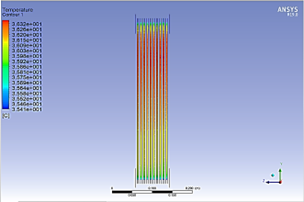(a)

## 6b.png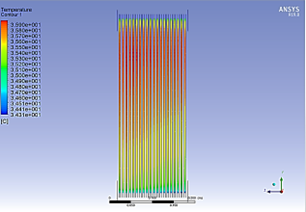(b)

## 6c.png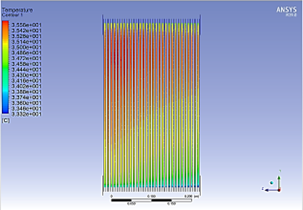(c)

The basic principle of heat exchangers is the transfer of heat energy, but not at the expense of the pressure value. It is necessary to maintain the pressure difference between the entry and exit area of the diaper on the value of the flow transfer of the fluid. Through Figure 7 it is noted that the value of the pressure difference decreases with the increase in the number of plates used in the heat exchanger, and that Because the flow is direct, the water is allowed to flow easily through the distributed pipe, where the value of the pressure difference in the case in which the number of plates is 30 was 0.35 pa, which is a small and serious pressure difference value compared to the other cases.

## 7a.png(a)

## 7b.png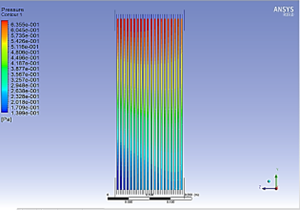(b)

## 7c.png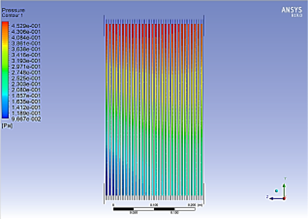(c)

5.2 The effect of the direction of flow on the value of heat transfer

The process of changing the direction of flow and making it take a longer path of movement and surface contact for the transfer of heat energy is necessary in plate heat exchangers. Through Figure 8, the method of heat transfer between the two types of flow notes that in the case of the reverse direction, it is better, as the exit temperature reached when the number of plates is 30, and in the reverse direction it was 302.889 K, which is better compared to the direct direction. Where he notes the way the temperature changes in the direct direction is from top to bottom, but in the other flow the heat transfer is from right to left.

## 8a.png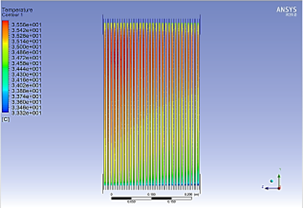(a)

## 8b.png(b)

Figure 8. Temperature contour of: (a) direct flow; (b) back and forth flow

The increase in the pressure difference in the heat exchangers is undesirable and unsatisfactory. As the case in which the one-way runs have a small pressure difference. But with the good transfer of thermal energy in the direction of the reverse flow, but in the same place, it has a high and undesirable pressure difference, as the pressure difference reached 734 Pa, as in Figure 9.

## 9a.png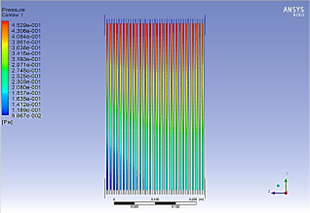(a)

## 9b.png(b)

Figure 9. Pressure contour of: (a) direct flow; (b) back and forth flow

Figure 10 shows the method of fluid flow and its obstruction in movement for the two types of flow, as it is noted that the values of velocity in the flow in the opposite direction are relatively more compared to the direct direction. The reason for this is that the path of movement it passes through is narrow.

## 10a.png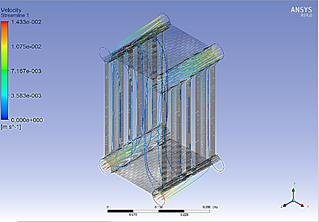(a)

## 10b.png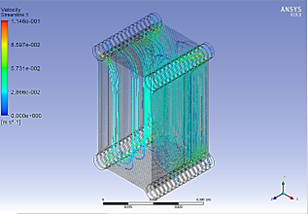(b)

Figure 10. Velocity stream line of: (a) direct flow; (b)back and forth flow

Table 1 and Figure 11 show the different parameters that were reached in the different cases and the strength of the reverse direction in the transfer of thermal energy with the large pressure generated as a result of this movement.

Table 1. The output result of CFD

 Number of blades Outlet temperature of direct case (K) Outlet temperature of back-and-forth case (K) Pressure difference of direct case (Pa) Pressure difference of back and forth case (Pa) 10 308.279 303.250 0.9462 237.912 20 308.104 303.004 0.4956 487.171 30 308.031 302.889 0.35623 735.597

## 11a.png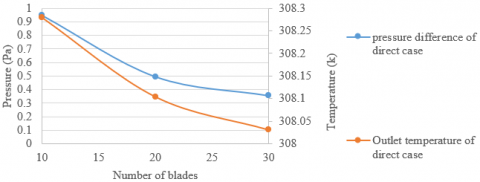(a)

## 11b.png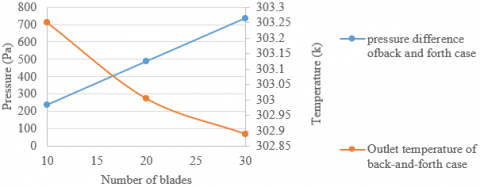(b)

Figure 11. Temperature and pressure difference of: (a) direct flow; (b)back and forth flow

6. Conclusion

The results will be summarized as follows:

1. Increasing the number of plates in the plate heat exchanger increases the transfer area and thus increases the value of the transferred heat energy. In the case where the best transfer of heat energy is compared to 20 and 10, where the exit temperature reached 308.104 K, we notice an increase in the transfer of thermal energy.
2. The basic principle of heat exchangers is the transfer of heat energy, but not at the expense of the pressure value. It is necessary to maintain the pressure difference between the entry and exit area of the fluid, rather than relying on the value of the flow transfer of the fluid. Because the flow is direct, the water is allowed to flow easily through the distributed pipe, where the temperature difference in the case in which the number of plates is 30 was 0.35 pa, a small and serious pressure difference value compared to the other cases.
3. The heat transfer between two different types of flow -one in the direct direction and the reverse direction- is achieved by changing the path of movement and surface contact for the transfer of heat energy. In a plate heat exchanger, the exit temperature reached when the number of plates is 30, and 302.889 K, which is better compared to the original direction.
4. The increase in the pressure difference in the heat exchangers is undesirable and unsatisfactory. As the one-way runs have a small pressure difference, but with the good transfer of thermal energy in the direction of the reverse flow, but in the same place, it has a high and undesirable pressure difference.
5. There are two types of flow -one in which the velocity in the flow in the opposite direction is relatively more compared to that in the direct direction. The reason for this is that the path of movement it passes through is narrow, and as a result, there is an obstruction in movement for it to pass through.
6. The many parameters attained in the various circumstances, as well as the intensity of the reverse direction in thermal energy transfer with the huge pressure created as a consequence of this movement.
Acknowledgement

The authors would like to thank Al-Mustaqbal University College for the assistance in completing this work.

References

 Chtourou, S., Djemel, H., Kaffel, M., Baccar, M. (2021). Predicting the effect of the rib pitch on thermal performance factor of small channels plate heat exchangers fitted with Y and C shapes obstacles. SN Applied Sciences, 3: 1-28. https://doi.org/10.1007/s42452-021-04473-z

 Li, W.Z., Hrnjak, P. (2021). Single-phase flow distribution in plate heat exchangers: Experiments and models. International Institute of Refrigeratio, 126: 45-56. https://doi.org/10.1016/j.ijrefrig.2021.01.026

 Elmekawy, A.M.N., Ibrahim, A.A., Shahin, A.M., Al-Ali, S., Hassan, G.E. (2021). Performance enhancement for tube bank staggered configuration heat exchanger – CFD Study. Chemical Engineering and Processing: Process Intensification, 164, Article ID: 108392. https://doi.org/10.1016/j.cep.2021.108392

 Kim, B.R., Kim, M.S., An, S.K., Park, C.W. (2020). Design of welded chevron plate heat exchanger: Application of recuperator in LiBr-water absorption systems. Journal of Mechanical Science and Technology, 34: 4763-4771. https://doi.org/10.1007/s12206-020-1033-3

 Eldeeb, R., Aute, V., Radermacher, R. (2020). Pillow plate heat exchanger weld shape optimization using approximation and parallel parameterized CFD and non-uniform rational B-splines. International Institute of Refrigeration, 110: 121-131. https://doi.org/10.1016/j.ijrefrig.2019.10.024

 Gürel, B., Akkaya, V.R., Göltaş, M., Şen, C.N., Güler, O.V., Koşar, M.L., Keçebaş, A.(2020). Investigation on flow and heat transfer of compact brazed plate heat exchanger with lung pattern. Applied Thermal Engineering: 175, Article ID: 115309. https://doi.org/10.1016/j.applthermaleng.2020.115309

 Taufiq, A., Dhakar, P.S. (2020). CFD analysis of plate heat exchanger by using ansys. International Journal Research and Analytical Reviews, 7(3). https://doi.org/10.13140/RG.2.2.16507.95526

 Dietrich, A., Nowitzki, M., Van De Sand, R., Reiff-Stephan, J. (2020). Numerical simulation of condensing ammonia in plate heat exchangers using CFD. European Council for Modelling and Simulation, 34: 41-47. https://doi.org/10.7148/2020-0041

 Alzahran, S., Islam, M.S., Saha, S.C. (2019). A thermo-hydraulic characteristics investigation in corrugated plate heat exchanger. Energy Procedia, 160: 597-605. https://doi.org/10.1016/j.egypro.2019.02.211

 Chien, N.B., Jong-Taek, O., Asano, H., Tomiyama, Y. (2019). Investigation of experiment and simulation of a plate heat exchanger. Energy Procedia, 158: 5635-5640. https://doi.org/10.1016/j.egypro.2019.01.575

 Shirzad, M., Delavar, M.A., Ajarostaghi, S.S.M., Sedighi, K. (2019). Evaluation the effects of geometrical parameters on the performance of pillow plate heat exchanger. Chemical Engineering Research Design, 150: 74-83. https://doi.org/10.1016/j.cherd.2019.06.032

 Emani, S., Ramasamy, M., Shaari, K.Z. (2019). Discrete phase-CFD simulations of asphaltenes particles deposition from crude oil in shell and tube heat exchangers. Applied Thermal Engineering, 149: 105-118. https://doi.org/10.1016/j.applthermaleng.2018.12.008

 Khanlari, A., Sözen, A., Variyenli, H. (2019). Simulation and experimental analysis of heat transfer characteristics in the plate type heat exchangers using TiO2/water nanofluid. International Journal of Numerical Methods for Heat & Fluid Flow, 29: 1343-1362. https://doi.org/10.1108/HFF-05-2018-0191

 Della Torre, A., Montenegro, G., Onorati, A., Khadilkar, S., Icarelli, R. (2019). Multi-scale CFD modeling of plate heat exchangers including offset-strip fins and dimple-type turbulators for automotive applications. Energies, 12(15): 2965. https://doi.org/10.3390/en12152965

 Schaer, N., Odinot, J.M., Tang, K., Dufresne, M., Vazquez, J., Isenmann, G. (2018). Numerical study of a plate heat exchanger using CFD: Comparison of texture loss assessed by rheological measurements. Journal Food Process Engineering, 41: 1-5. https://doi.org/10.1111/jfpe.12889

 Bhattad, A., Sarkar, J., Ghosh, P. (2018). Discrete phase numerical model and experimental study of hybrid nanofluid heat transfer and pressure drop in plate heat exchanger. International Communications in Heat and Mass Transfer, 91: 262-273. https://doi.org/10.1016/j.icheatmasstransfer.2017.12.020

 Lazarevikj, M., Filkoski, R.V., Markov, Z. (2018). CFD analysis of heat transfer and fluid flow in plate heat exchanger with nanofluid. Conference in 3rd South East European Conference on Sustainable Development of Energy, Water and Environment Systems - SDEWES SEE, Novi Sad, Serbia.

 Mandic, D. (2020). CFD modeling of turbulence in channels of plate heat exchangers. Journal of Fluid Flow, Heat and Mass Transfer, 7: 46-57. https://doi.org/10.11159/jffhmt.2020.005

 Abed, A.M., Abed, I.A., Majdi, H.S., Al-Shamani, A.N., Sopian, K. (2016). A new optimization approach for shell and tube heat exchangers by using electromagnetism-like algorithm (EM). Heat Mass Transfer, 52: 2621-2634. https://doi.org/10.1007/s00231-016-1769-6

 Majdi, H.S., Alabdly, H.A., Hasan, B.O., Hathal, M.M. (2019). Oil fouling in double-pipe heat exchanger under liquid-liquid dispersion and the influence of copper oxide nanofluid. Heat Transfer—Asian Research, 48: 1963-1981. https://doi.org/10.1002/htj.21473

 Hasan, B.O., Majdi, H.S., Hathal, M.M. (2020). Study on oil fouling in a double pipe heat exchanger with mitigation by a surfactant. Heat Transfer, 49: 2645-2658. https://doi.org/10.1002/htj.21738

 Mahmoud, A. Mashkour, Hasan Shakir Majdi, Laith Jaafer Habeeb. (2021). Enhancement of forced convection heat transfer in tubes and heat exchangers using passive techniques: A review. Journal of Mechanical Engineering Research and Developments, 44(3): 208-218.

 Tarrad, A.H. (2021). Borehole thermal analysis for a closed loop vertical U-tube DX ground heat exchanger. Mathematical Modelling of Engineering Problems, 8(4): 501-509. https://doi.org/10.18280/mmep.080402

 Abbas, A.S., Mohammed, A.A. (2022). Augmentation of plate-fin heat exchanger performance with support of various types of fin configurations. Mathematical Modelling of Engineering Problems, 9(5): 1406-1414. https://doi.org/10.18280/mmep.090532